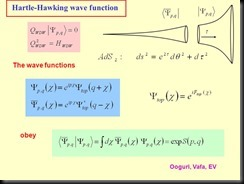# The reality of the wave function.

There are two ways science attempts to explain and define the behavior of our universe. The first is Quantum mechanics or the branch of physics defines its evolution in terms of the probabilities associated with the wave function. The other is the deterministic universe of Einstein which defines it in terms of a physical interaction between space and time.

Specifically, Einstein defined the position a particle in terms of where an increase in the energy density in space associated with its mass is located.

While quantum mechanics uses the mathematical interpretation of the wave function to define the most probable position of a particle when observed.Since we all live in the same world you would expect the probabilistic approach of quantum mechanics to be compatible with the deterministic one of Einstein. Unfortunately, they define two different worlds which appear to be incompatible. One defines existence in terms of the probabilities while the other defines it in terms of the deterministic of properties of space and time.

However, even though those probabilities appears to be incompatible with Relativity’s determinism they can be explained in terms of a physical interaction between space and time.

For example, when we role dice in a casino most of us realize the probability of a six appearing is related to or is caused by its physical interaction with properties of the table in the casino where it is rolled. Putting it another way what defines the fact that six appears is NOT the probability of getting one but the interaction of the dice with the table and the casino it occupies.

Therefore, to integrate the probabilistic interpretation of the wave function in terms of the deterministic properties of space and time one must show how and why an interaction between them is responsible for the position of a particle when observed .

One way of doing this is to use the fact that evolution in both a quantum and space-time environments are controlled by a wave.

For example, Relativity defines evolution of space-time in terms of the energy propagated by electromagnetic wave while Quantum Mechanics defines it in terms of the mathematical evolution of the wave function.

This suggests the wave function that governs the evolution of a quantum environment may be a mathematical representation of an electromagnetic wave that governs evolution in the world of Relativity.  If true one should be able to derive the probabilistic predictions of quantum mechanics in terms of the deterministic properties of an electromagnetic wave in space-time.

For example, the science of wave mechanics along with the fact Relatively tells us electromagnetic moves continuously through space-time unless it is prevented from doing so by someone observing or something interacting with it. This would result in its energy being confined to three-dimensional space. The science of wave mechanic also tells us the three-dimensional “walls” of this confinement will result in its energy being reflected back on itself thereby creating a resonant or standing wave in three-dimensional space. This would cause its wave energy to COLLAPSE and concentrated at the point in space were a particle would be found. Additionally, wave mechanics also tells us the energy of a resonant system, such as a standing wave can only take on the discrete or quantized values associated with its fundamental or a harmonic of its fundamental frequency. This means a particle would occupy an extended volume of space defined by the wavelength of its standing wave.

Putting it another way what defines the fact that a particle appears where it does is NOT determined by the probabilities associated with the wave function but the deterministic interaction of an electromagnetic wave with the physical properties of space-time

However, the probabilistic interpretation of the wave function is required because Quantum mechanics defines the position of a particle in terms of mathematical point in space which it randomly defines respect to its center. Therefore, the randomness of where that point is with respect to a particle’s center will result in its position, when observed to be randomly distributed in space. This means one must define where it appears in terms of probabilities to average the deviations that are caused by the random placement of that point.

This shows why Relativity is indeterminate on a quantum scale while being deterministic on a macroscopic level because those deviations would be averaged out by the large number of particles in objects like the moon and planets

It shows how one can derive the “Reality” of probabilistic world of quantum mechanics in terms of the deterministic one of space-time by assuming the wavefunciton is a mathematical representation of an electromagnetic wave.